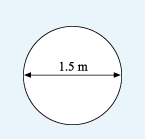Science, Maths & Technology

### Become an OU studentSucceed with maths: part 2

Start this free course now. Just create an account and sign in. Enrol and complete the course for a free statement of participation or digital badge if available.

# 2.2 Using π (pi)

If you could measure these objects more accurately, you would find that the circumference divided by the diameter always gives the same answer. This value is known as ‘pi’ (pronounced ‘pie’) and it is denoted by the Greek letter . The value of is approximately 3.142, although for most calculations you will be using the button on your calculator, which shows pi to many more decimal places.

In fact, pi is an irrational number. This means that it can’t be represented exactly as a fraction and has an infinite number of decimal places, with no repeating pattern of numbers.

This knowledge that the circumference divided by the diameter for any circle gives a constant value of around 3.142 enables a formula to calculate the circumference from the diameter or the radius of a circle to be formed. Therefore:

Because the diameter is twice the radius, this can also be written as:

You will now be asked to select the relevant formula to use in the next activity.

## Activity 4 Circumference of a circle

Timing: Allow approximately 5 minutes

Suppose a circular table has a diameter of 1.5 metres. How many people can sit down comfortably for a meal at the table, assuming that each person requires a space of about 0.75 m? Use the button marked with π on your calculator. If you need a hint or tip simply click on ‘reveal comment’.Figure 14 Diagram of a circular table with a 1.5 m diameter

### Comment

If you want some help to visualise the problem you could draw a quick sketch of the table and gaps for each person.